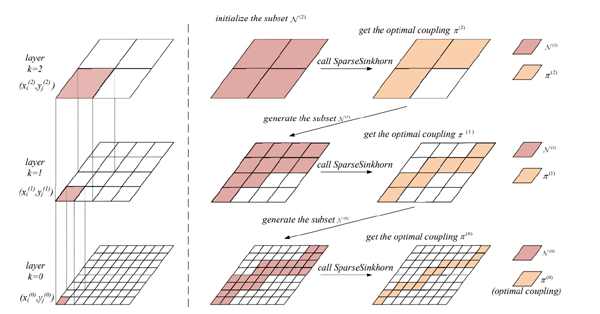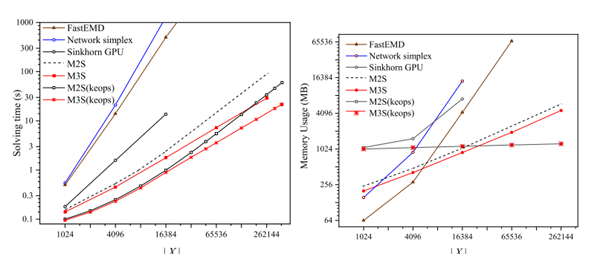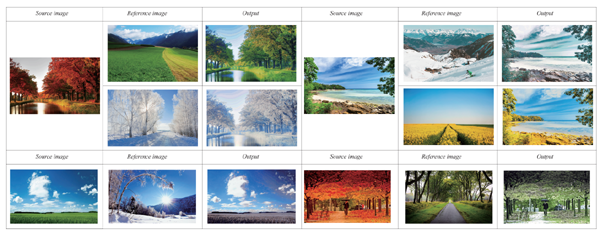Location:Home >> Research >> Research Progress

## The High-Performance Computing Department Makes Progress in Large-Scale Computational Optimal Transport

Date: Mar 16, 2022

Recently, for the problem of computing optimal transport between discrete measures, CHEN Yidong, a PhD student in the Department of High Performance Computing, with the support of Prof. LU Zhonghua, proposed a Multi-scale Sparse Sinkhorn's algorithm to compute the optimal transport between discrete measures. The proposed algorithm is implemented and optimized in the unified Computing Device Architecture (CUDA) and applied to color transfer between large-size images. This research result was accepted by IEEE Conference on Computer Vision and Pattern Recognition (CVPR, CCF A).

Optimal transport is used to show the similarity of two distributions, which plays an important role in finance, statistics, physics, and machine learning. Computing the optimal transport between probability distributions is very hot in algorithm research. Using the commercial solver Cplex to calculate the optimal transport between two images with 256*256 pixels takes several hours and consumes nearly 100GB of memory.

Based on this problem, the researchers proposed the algorithm for iterating over sparse subsets and propose a multi-scale method to estimate sparse subsets. By constructing the Lyapunov function, we obtain the upper bound estimate of the convergence rate of the proposed algorithm, and the upper bound of truncation parameter is given for the first time, which is the best theoretical result so far. By implementing and optimizing the algorithm on CUDA, the computing time of optimal transmission between 256*256 images is reduced to less than 3 seconds, and the memory consumption is less than 3GB, which achieves more than 1000 times faster than commercial solvers and memory consumption is 30 times less.Figure 1. Estimating the subset by the multi-scale StructureFigure 2. Running time and memory requirement of different algorithms.Figure 3. Color transfer between 1920×1200 images.